# An elliptic remark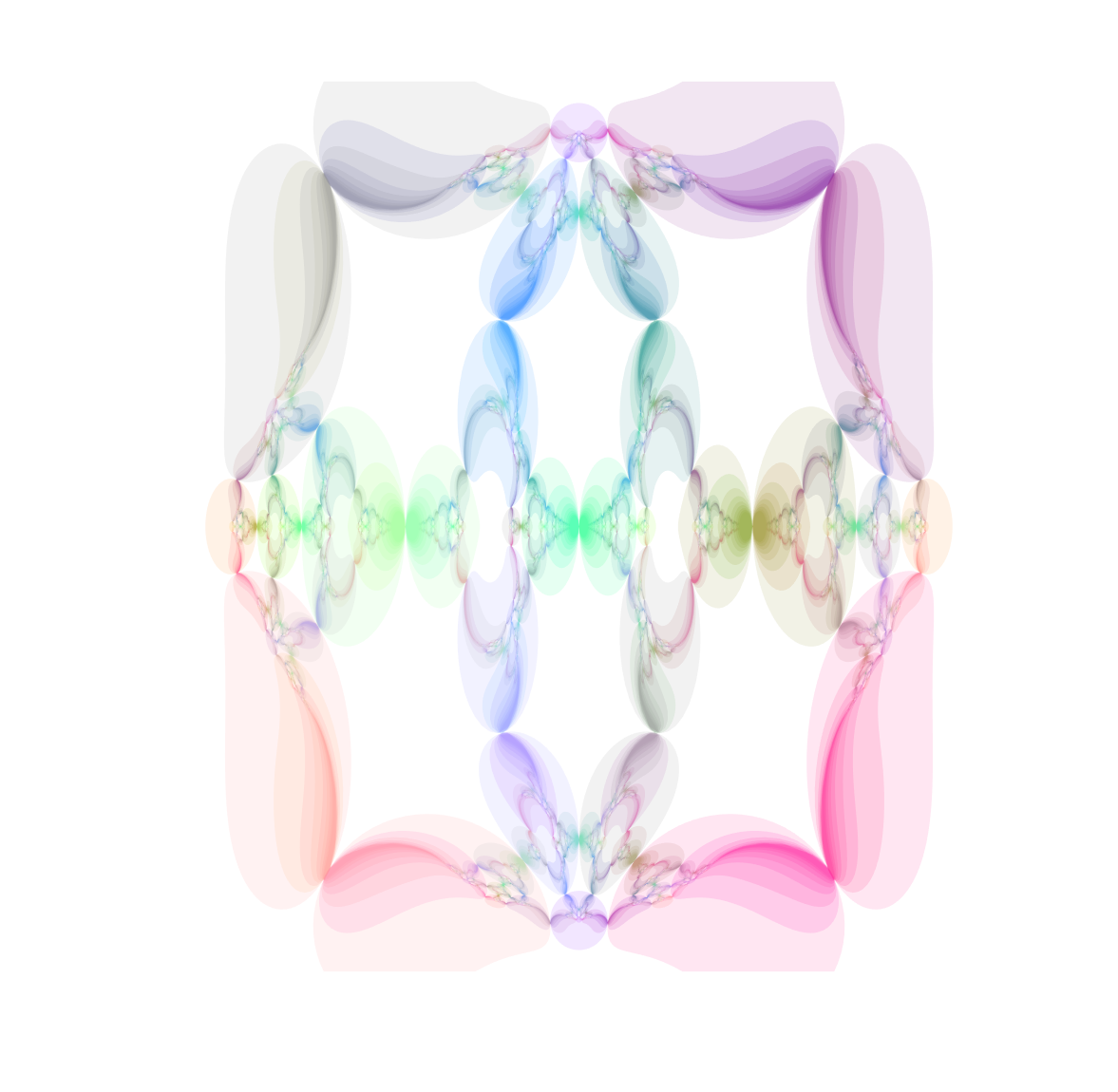I recently returned to toying around with circle and sphere inversion fractals, that is, fractal sets that are invariant under inversion in a given set of circles or spheres.

That got me thinking: can you invert points in other things than circles? Of course you can! José L. Ramírez has written a nice overview of inversion in ellipses. Basically a point$P$ is projected to another point$P'$ so that$||P-O||\cdot ||P'-O||=||Q-O||^2$ where$O$ is the centre of the ellipse and$Q$ is the point where the ray between$O, P', P$ intersects the ellipse.

In Cartesian coordinates, for an ellipse centered on the origin and with semimajor and minor axes$a,b$, the inverse point of$P=(u,v)$ is$P'=(x,y)$ where$x=\frac{a^2b^2u}{b^2u^2+a^2v^2}$ and$y=\frac{a^2b^2v}{b^2u^2+a^2v^2}$. Basically this is a squashed version of the circle formula.

Many of the properties remain the same. Lines passing through the centre of the ellipse are unchanged. Other lines get mapped to ellipses; if they intersect the inversion ellipse the new ellipse also intersect it at those points. Hence tangent lines are mapped to tangent ellipses. Ellipses with parallel axes and equal eccentricities map onto other ellipses (or lines if they intersect the centre of the inversion ellipse). Other conics get turned into cubics; for example a hyperbola gets mapped to a lemniscate. (See also this paper for more examples).

Now, from a fractal standpoint this means that if you have a set of ellipses that are tangent you should expect a fractal passing through their points of tangency. Basically all of the standard circle inversion fractals hence have elliptic counterparts. Here is the result for a ring of 4 or 6 mutually tangent ellipses: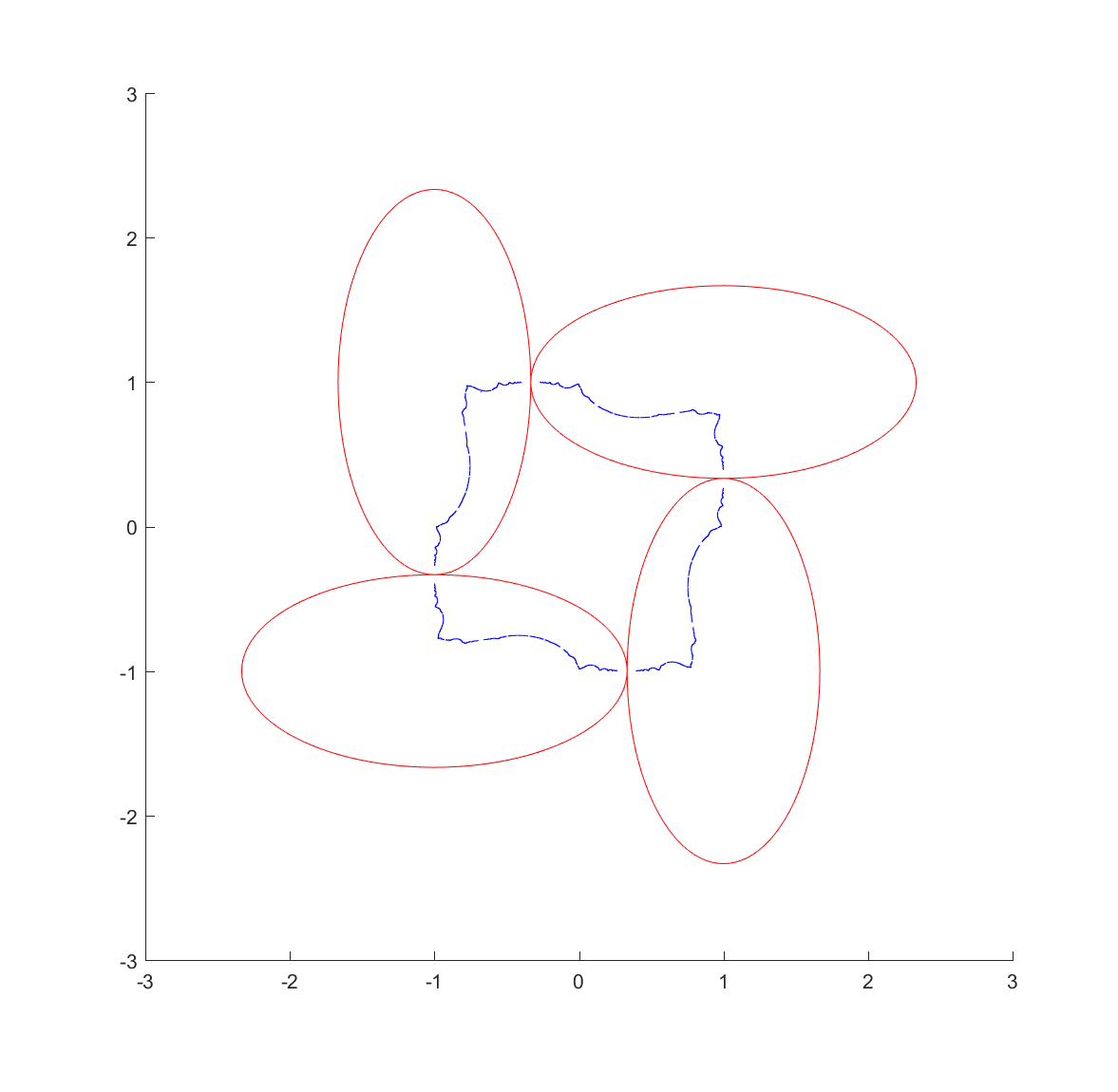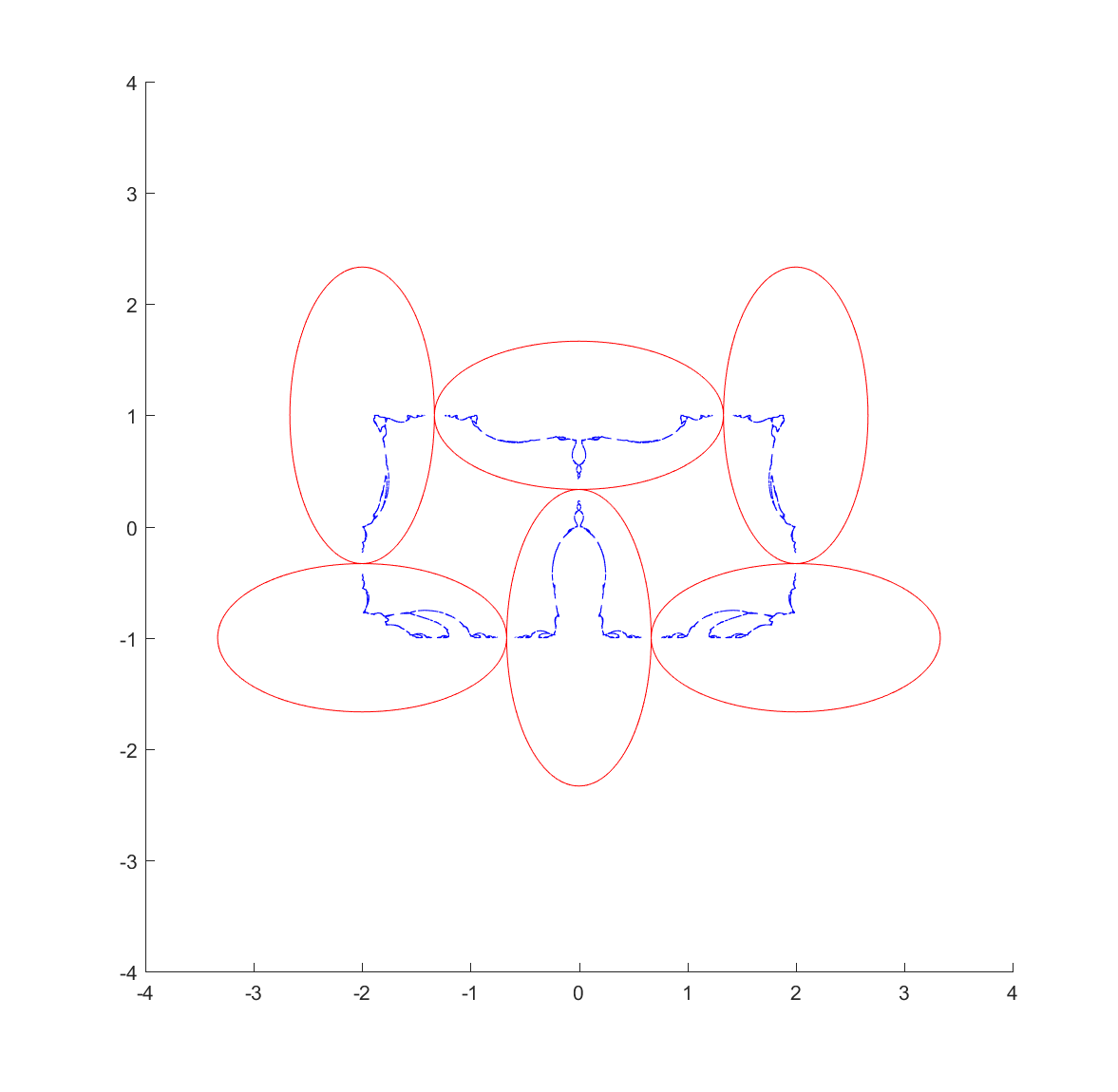Invariant set fractal (blue) for inversion in the red ellipses. Generated using an IFS algorithm.

These pictures were generated by taking points in the plane and inverting them with randomly selected ellipses; as the process continues they get attracted to the invariant set (this is basically a standard iterated function system). It also has the known problem of finding the points at the tangencies, since the iteration has to loop consistently between inverting in the two ellipses to get there, but it is likely that a third will be selected at some point.

One approach is to deliberately recurse downward to find the points using a depth first search. We can take look at where each ellipse is mapped by each of the inversions, and since the fractal is inside each of the mapped ellipses we can then continue mapping the chain of mapped ellipses, getting nice bounds on where it is going (as long as everything is shrinking: this is guaranteed as long as it is mappings from the outside to the inside of the generating ellipses, but if they were to overlap things can explode). Doing this for just one step reveals one reason for the quirky shapes above: some of the ellipses get mapped into crescents or pears, adding a lot of bends: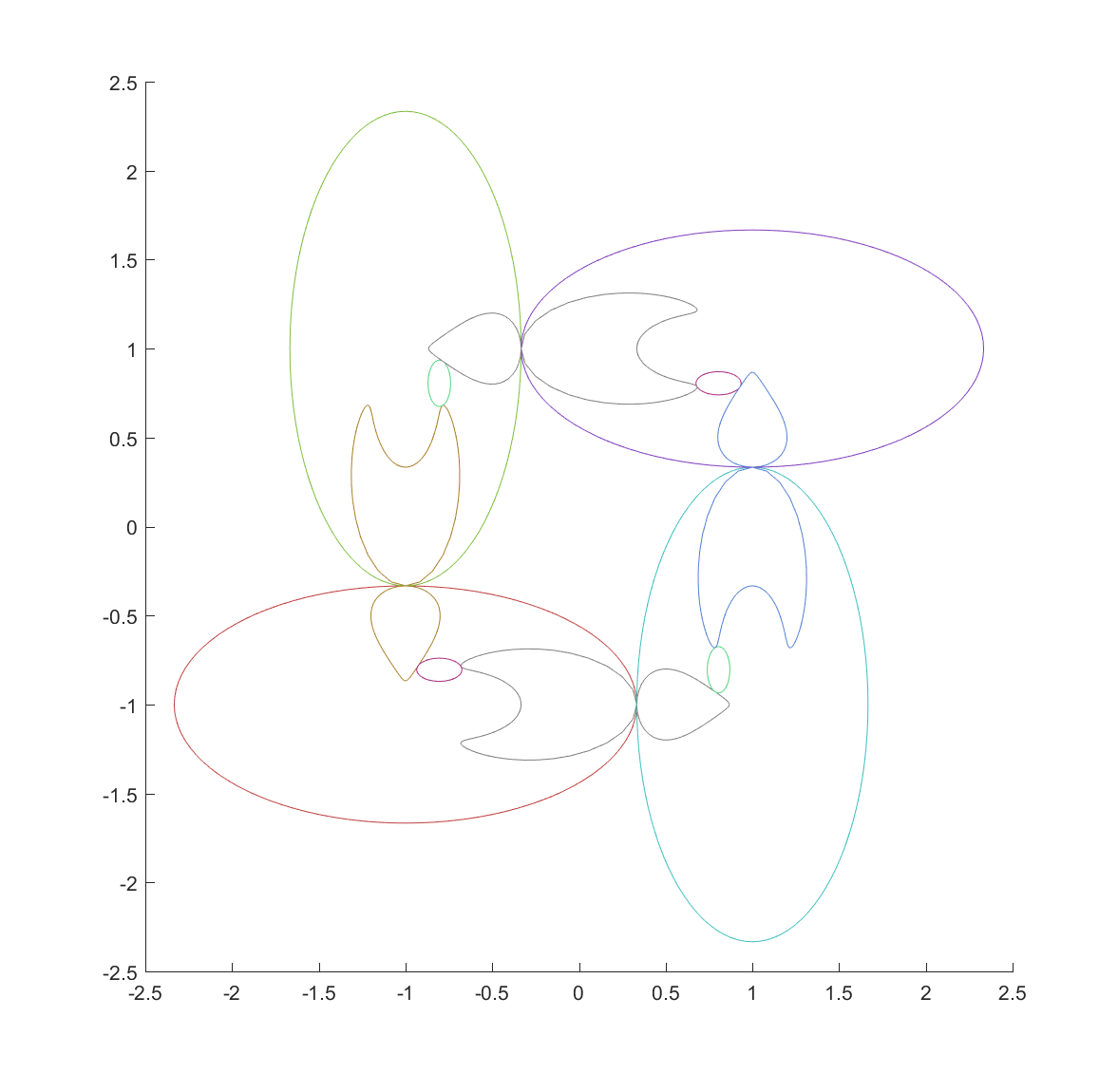Mappings of the ellipses by their inversions: each of the four main ellipses map the other three to their interior but distort the shape of two of them.

Now, continuing this process makes a nested structure where the invariant set is hidden inside all the other mapped ellipses.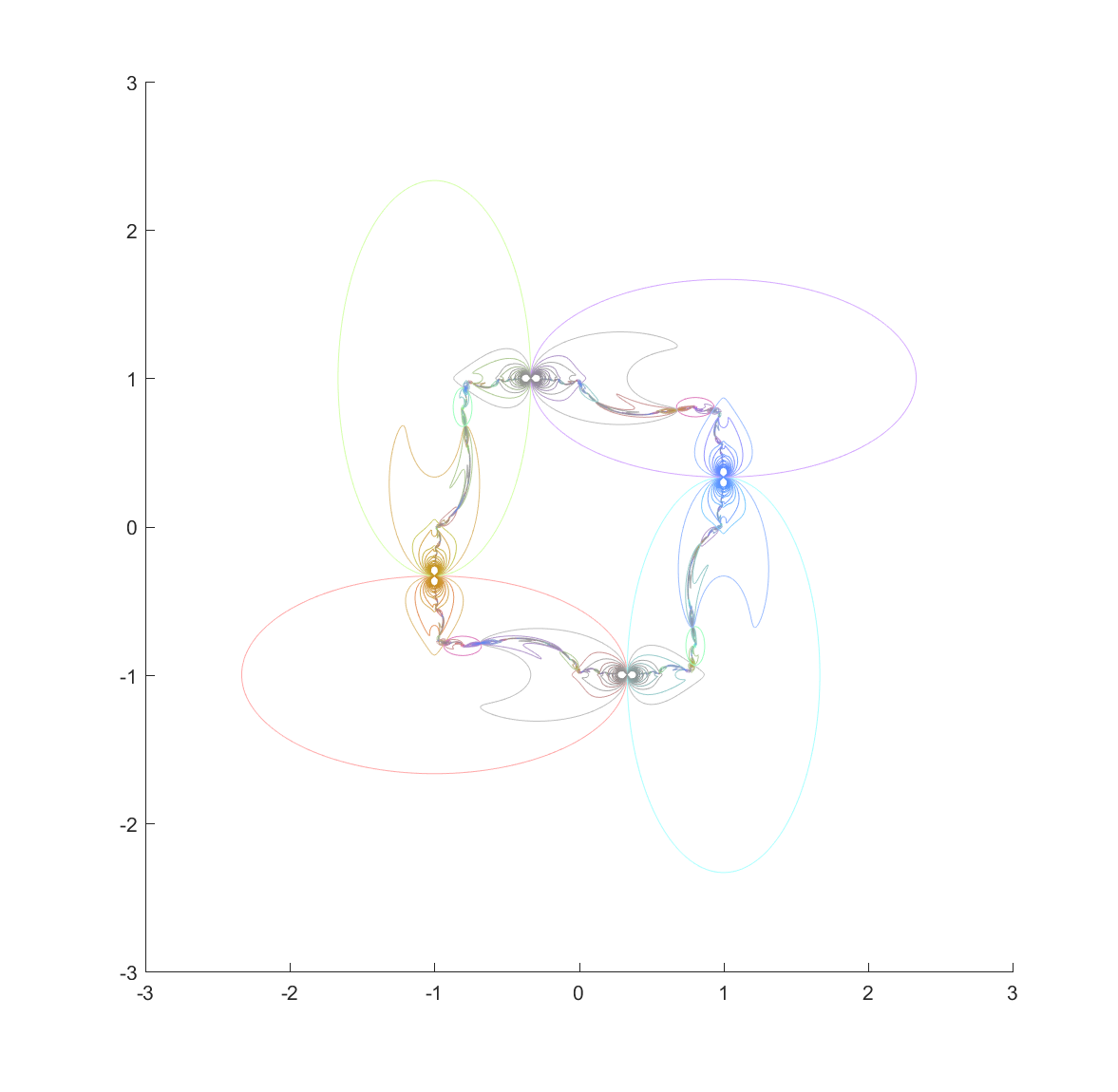Nested mappings of the ellipses in the chain, bounding the invariant set. Colors are mixtures of the colors of the generating ellipses, with an increase in saturation.

It is still hard to reach the tangent points, but at least now they are easier to detect. They are also numerically tough: most points on the ellipse circumferences are mapped away from them towards the interior of the generating ellipse. Still, if we view the mapped ellipses as uncertainties and shade them in we can get a very pleasing map of the invariant set: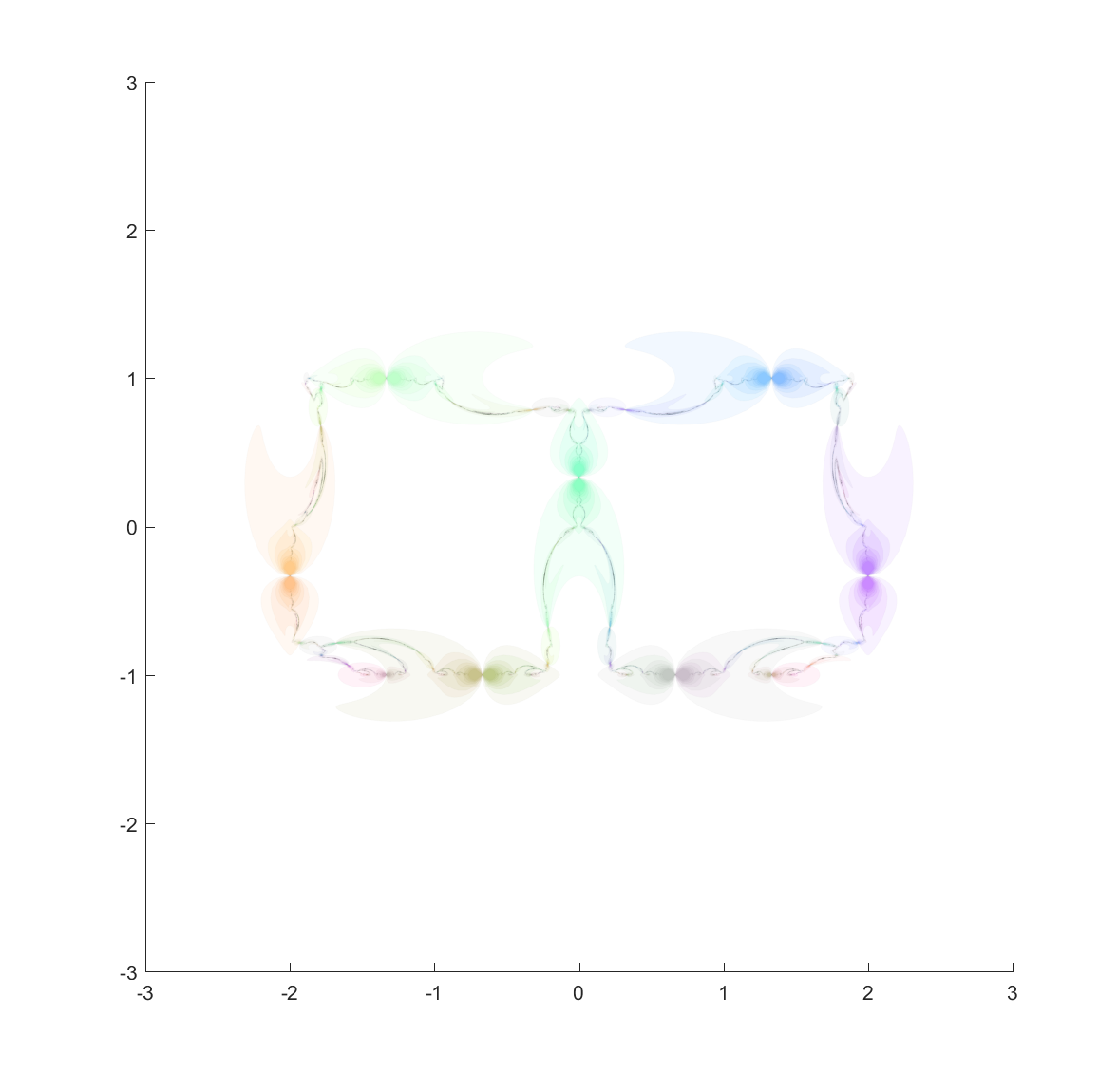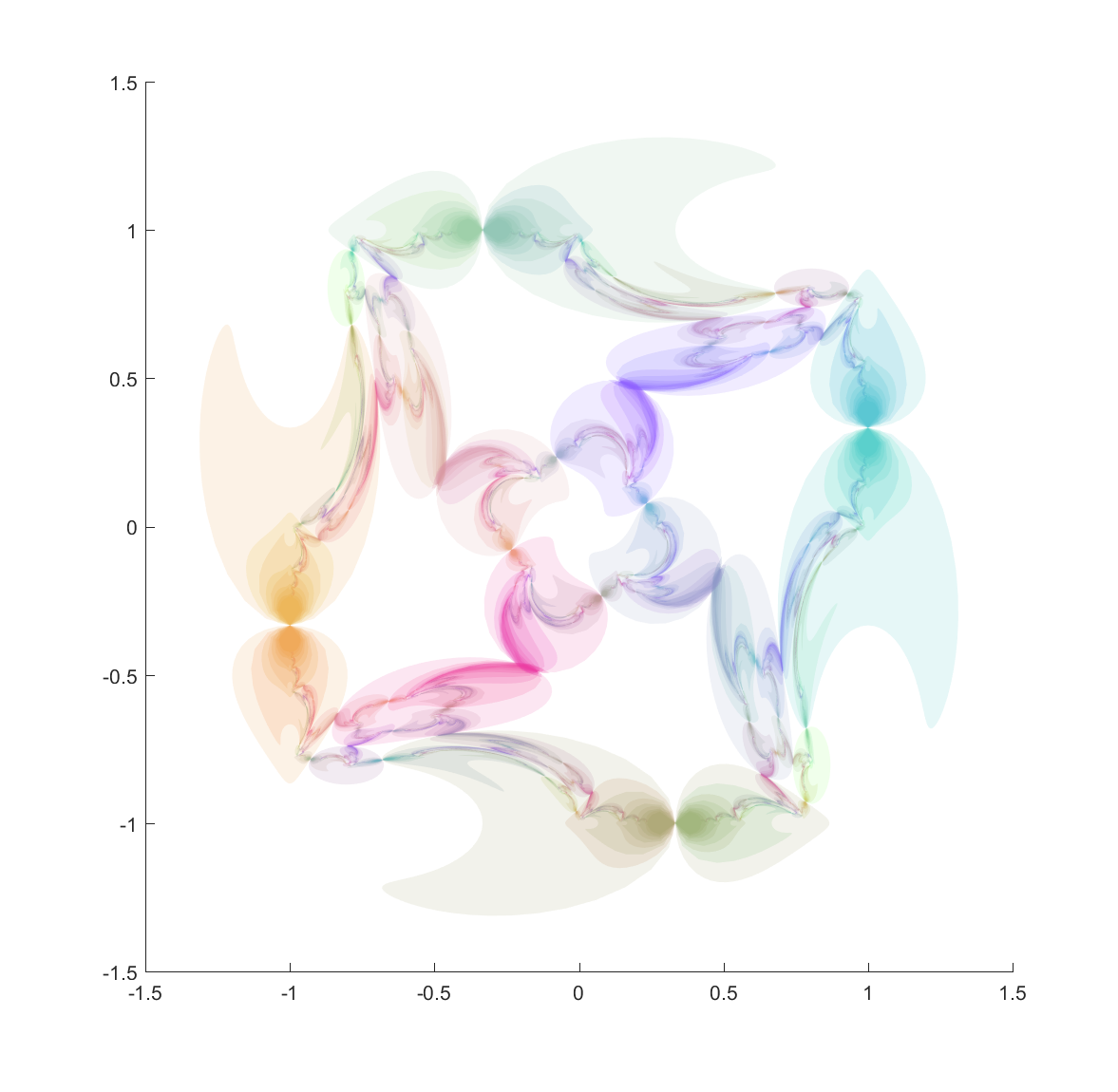Invariant set of chain of four outer ellipses and a circle tangent to them on the inside.

Here are a few other nice fractals based on these ideas: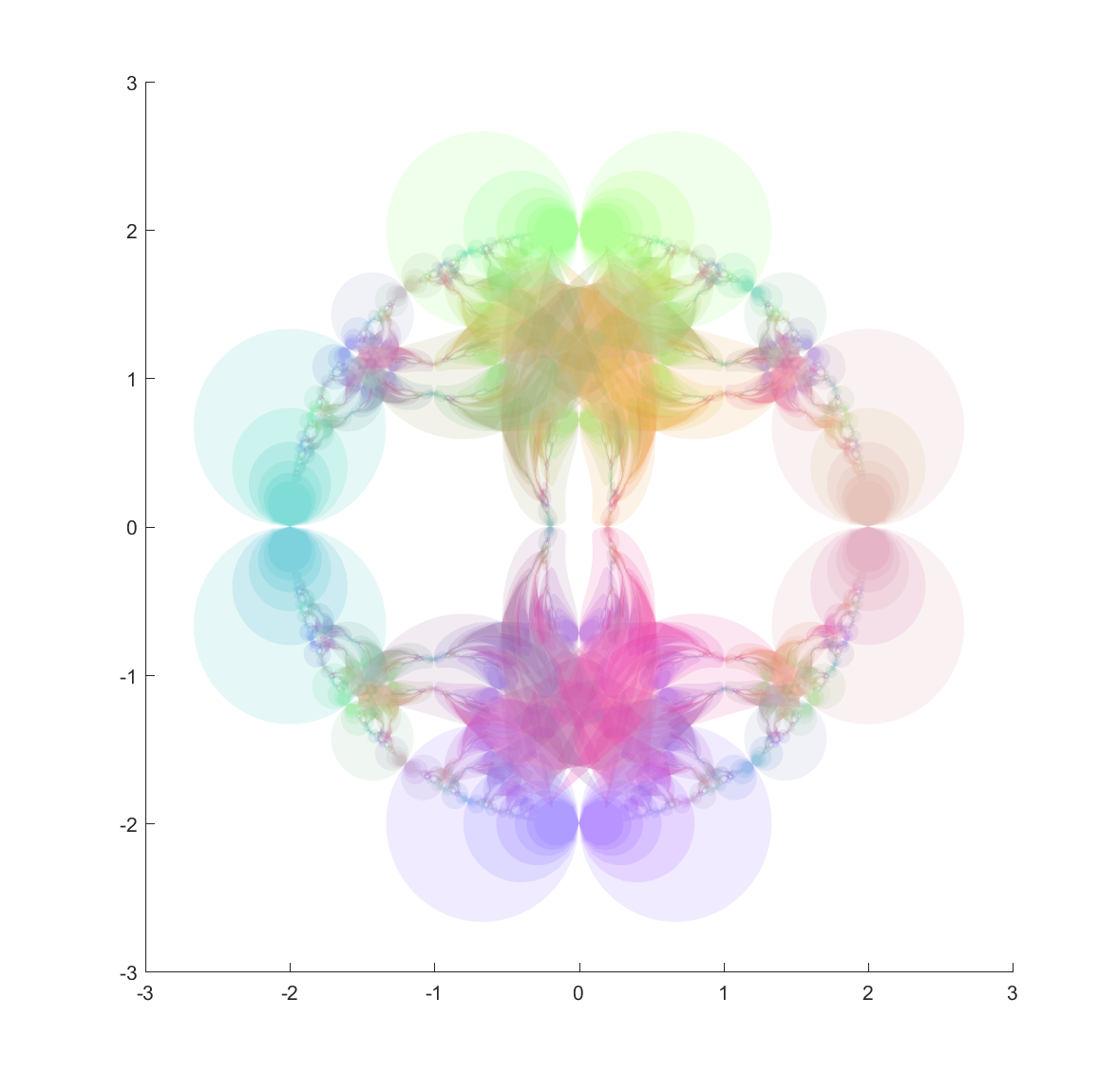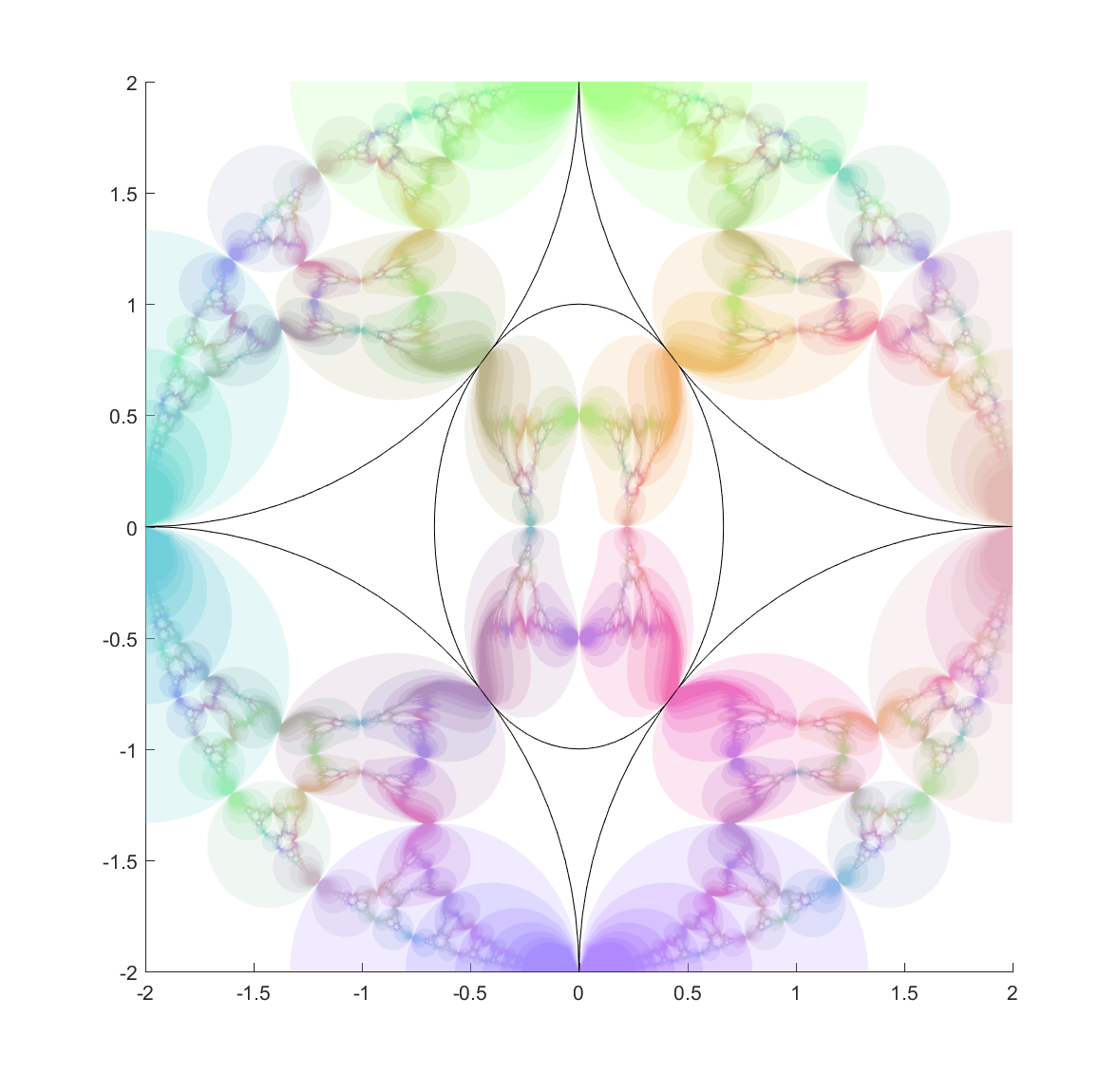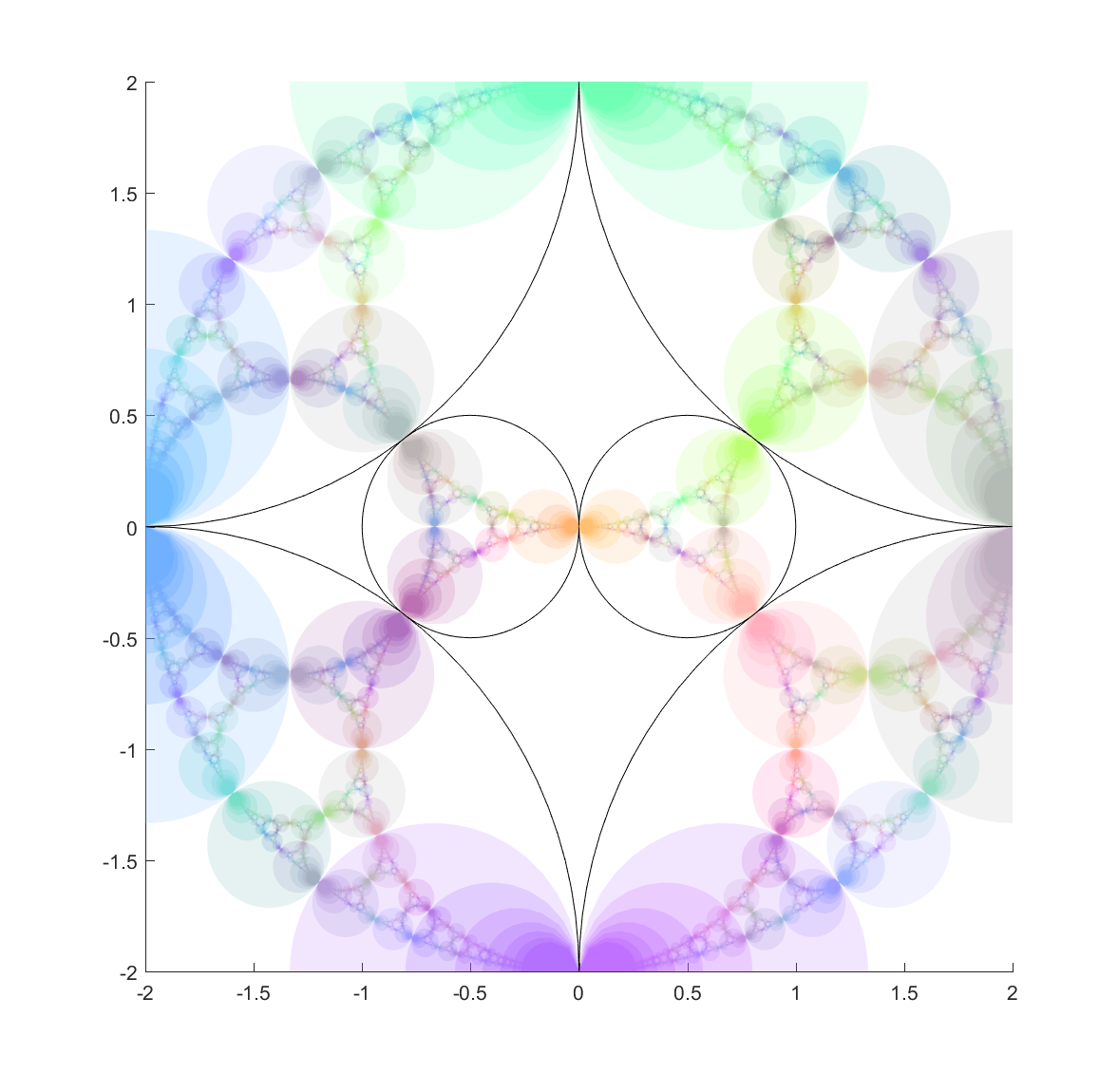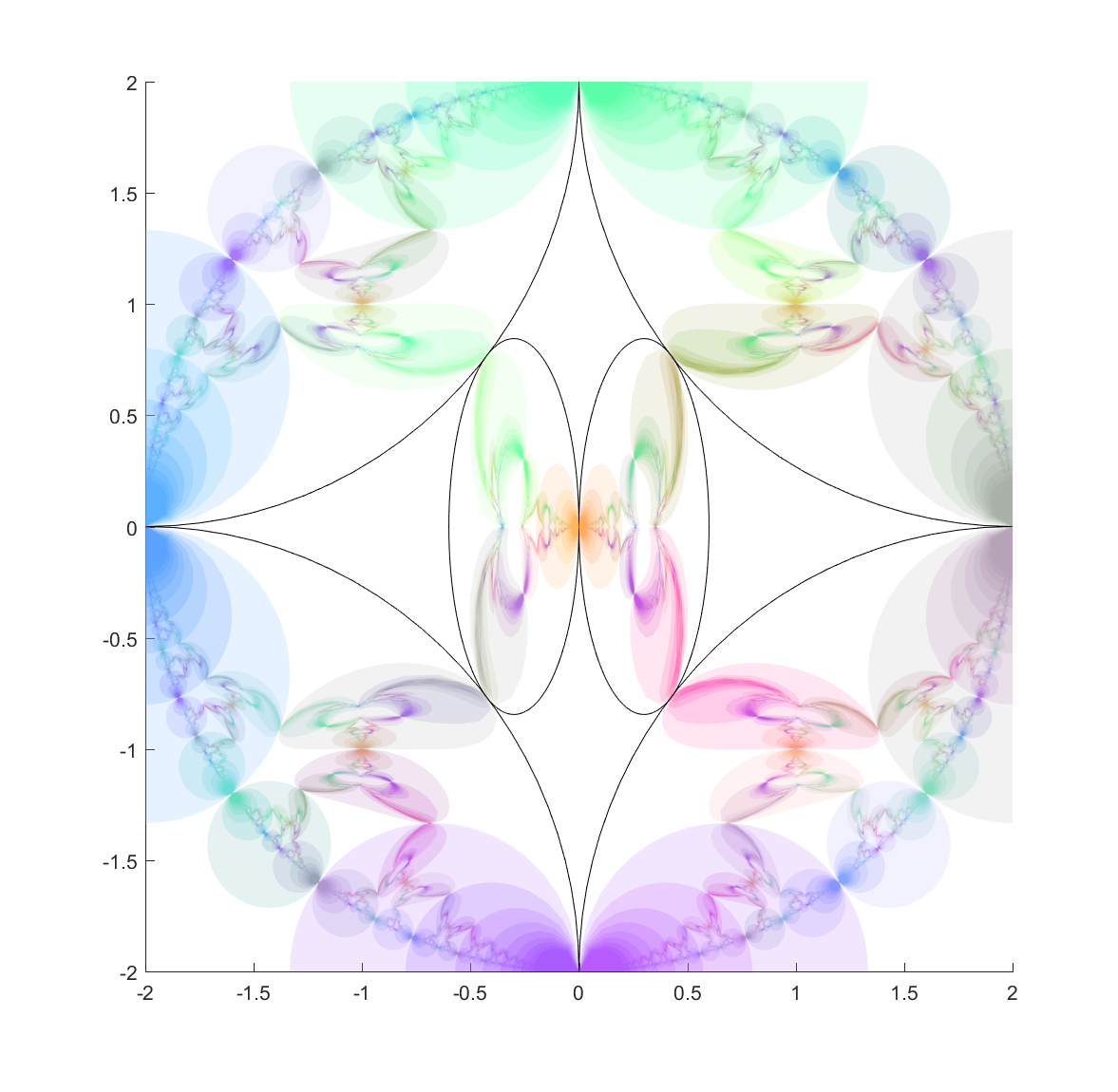Using a mix of circles and ellipses produces a nice mix of the regularity of the circle-based Apollonian gaskets and the swooshy, Hénon fractal shape the ellipses induce.

# Appendix: Matlab code

% center=[-1 -1 2 1; -1 1 1 2; 1 -1 1 2; 1 1 2 1];
% center(:,3:4)=center(:,3:4)*(2/3);
%
%center=[-1 -1 2 1; -1 1 1 2; 1 -1 1 2; 1 1 2 1; 3 1 1 2; 3 -1 2 1];
%center(:,3:4)=center(:,3:4)*(2/3);
%center(:,1)=center(:,1)-1;
%
% center=[-1 -1 2 1; -1 1 1 2; 1 -1 1 2; 1 1 2 1];
% center(:,3:4)=center(:,3:4)*(2/3);
% center=[center; 0 0 .51 .51];
%
% egg
% center=[0 0 0.6666 1; 2 2 2 2; -2 2 2 2; -2 -2 2 2; 2 -2 2 2];
%
% double
%r=0.5;
%center=[-r 0 r r; r 0 r r; 2 2 2 2; -2 2 2 2; -2 -2 2 2; 2 -2 2 2];
%
% Double egg
center=[0.3 0 0.3 0.845; -0.3 0 0.3 0.845; 2 2 2 2; -2 2 2 2; -2 -2 2 2; 2 -2 2 2];
%
M=size(center,1); % number of ellipses
N=100; % points on fill curves
X=randn(N+1,2);
clf
hold on
tt=2*pi*(0:N)/N;
alpha 0.2
for i=1:M
X(:,1)=center(i,1)+center(i,3)*cos(tt);
X(:,2)=center(i,2)+center(i,4)*sin(tt);
plot(X(:,1),X(:,2),'k');
for j=1:M
if (i~=j)
recurseDown(X,[i j],10,center)
drawnow
end
end
end


## Recursedown.m

function recurseDown(X,ellword,maxlevel,center)
i=ellword(end); % invert in latest ellipse
%
% Perform inversion
C=center(i,1:2);
A2=center(i,3).^2;
B2=center(i,4).^2;
Y(:,1)=X(:,1)-C(:,1);
Y(:,2)=X(:,2)-C(:,2);
X(:,1)=C(:,1)+A2.*B2.*Y(:,1)./(B2.*Y(:,1).^2+A2.*Y(:,2).^2);
X(:,2)=C(:,2)+A2.*B2.*Y(:,2)./(B2.*Y(:,1).^2+A2.*Y(:,2).^2);
%
if (norm(max(X)-min(X))<0.005) return; end
%
co=hsv(size(center,1));
coco=mean([1 1 1; 1 1 1; co(ellword,:)]);
%
%    plot(X(:,1),X(:,2),'Color',coco)
fill(X(:,1),X(:,2),coco,'FaceAlpha',.2,'EdgeAlpha',0)
%
if (length(ellword)<maxlevel)
for j=1:size(center,1)
if (j~=i)
recurseDown(X,[ellword j],maxlevel,center)
end
end
end


## One thought on “An elliptic remark”

1.Christof Baumgartner says:

Great work, I really like it